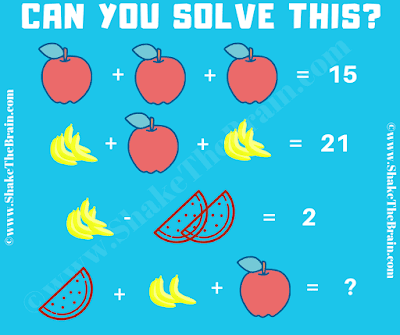## Tuesday, October 27, 2020

In this Fruits Math Picture Puzzle, your challenge is to solve the given math equations and find the values of the given fruits. In this Math Picture Puzzle, you are given some algebraic equations. Solve these math equations, to find values of the individual fruit and then solve the last equation to find the value of the missing number which will replace the question mark.Can you solve this Math Picture Puzzle?
The answer to this "Fruits Math Picture Puzzle", can be viewed by clicking on the answer button.

1.Incorrect
Yes each banana is 2, that means the watermelon is 3.

1.You are right. I have corrected the answer to this fruits math puzzle. However the answer is 14.

2.umm i dont understand...i got apple = 5, each banana = 2 , watermelon = 6
hence watermelon + 3 bananas + apple = 6 + 6 + 5 = 17

3.nevermind....now i understand